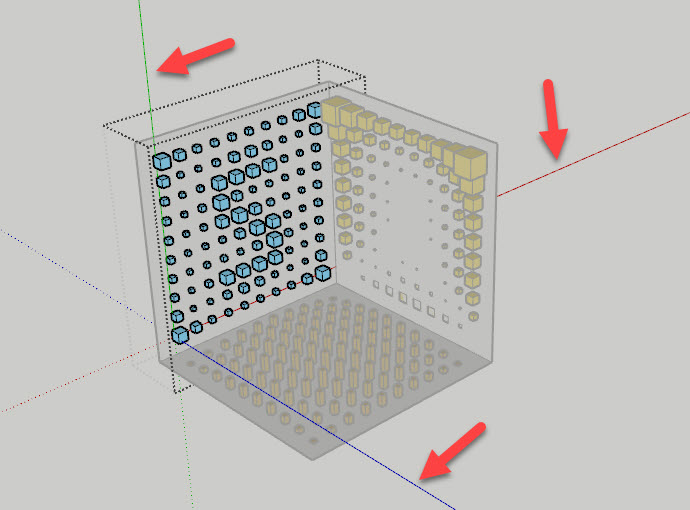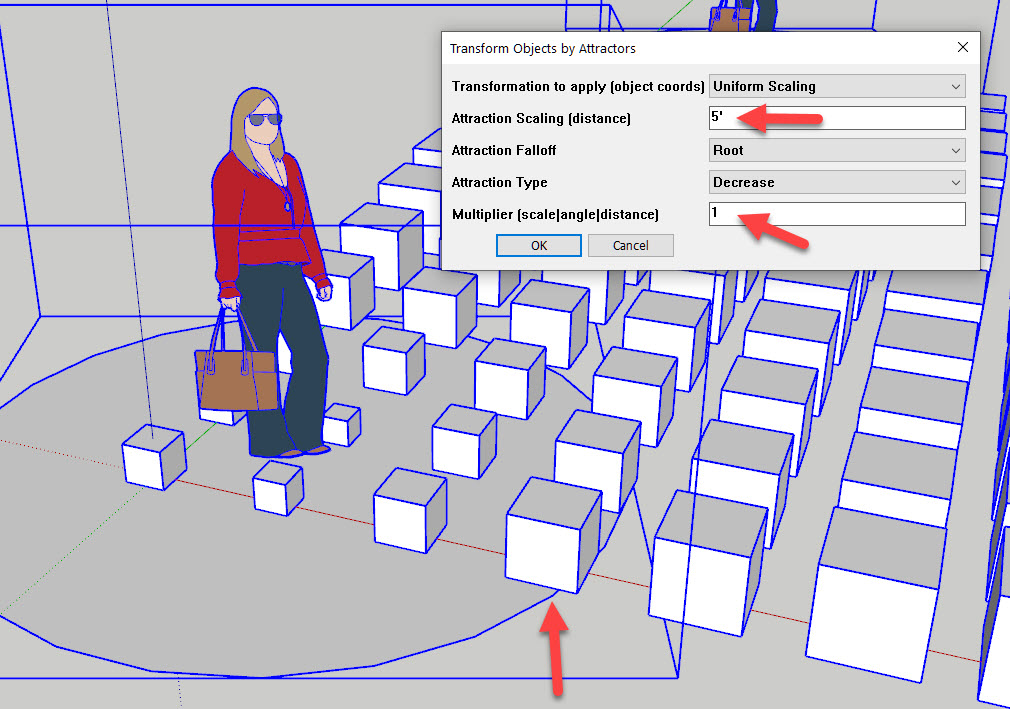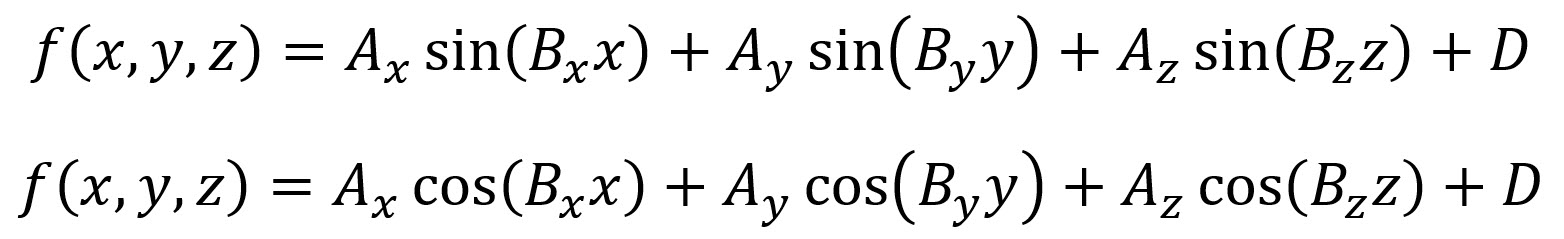# Scale By Tools Manual

The following is a reference manual for the latest version of the Scale By Tools for SketchUp. It is assumed that you have successfully installed this extension (instructions are on the previous page).

## Axes

It is important to remember that each of these tools operates in a “local” coordinate context since selected objects will be temporarily grouped while the tool works on them. This approach is very useful especially when you have modified axes or non-orthogonally arranged objects.

The image below shows how a typical local coordinate system looks like. Basically, any reference to each of the axes (red/green/blue) in the tools references the local coordinate system that gets created when you group the selected items (instead of the main, unmodified SketchUp coordinate system). In the image below, you can see that the “s” set of boxes resides on the red-green plane. Therefore, when you use an image to scale items, you need to select the “red-green” option for its alignment to get the correct result.While these tools should work well with any set of objects, SketchUp has a built-in logic with respect to the axes when it creates a group. Therefore, if your results don’t look as expected, I would advise to first group all objects so that you can go into group edit mode and then see how the axes are arranged. Then just select all objects inside the group and run the tool.

Any of the transformations (scaling / rotation / move) work on groups and components as follows:

• For a group, they are based on the group’s bounding box center. This is often the “center” of the object.
• For a component instance, they are based on the insertion point (it’s internal origin). This is often the “base” of the object (see image below).

You can control performance by carefully placing a component’s internal axes. Also keep in mind that the transformations are based on that internal coordinate system. This means that a “scaling in blue” will make the object taller, independent of its global orientation.## Image-Based Tools

Since the image-based tools rely on SketchUp’s `ImageRep` feature, which was introduced in the 2018 version, older versions of SketchUp can’t use these tools. The equation- and attractor-based tools will still work, however.

When working with images, remember that this tool only uses greyscale values. It will convert color data, however, so there’s no need to convert images first. Some tips for images:

• Adjust contrast and brightness to control scaling magnitudes
• If you want to achieve maximum scaling values, reduce your image to only black and white (no greyscale)
• Invert an image if you want to control its scaling direction
• Apply a gaussian blur to an image to get smoother transitions

## Attractor-Based Tools

You can define the attractor(s) by simply renaming a component in your model (e.g. the scale figure here) as “A”. When selected, all copies of that component will then be used to modify all other selected objects.To illustrate the effect of the scaling distance and the multiplier parameter, I added a circle with a 5′ radius under the attractor-figure in the image below. As you can see, the multiplier value will be reached at the scaling distance. The highlighted box at the bottom of the image has approximately its original size while those closer to the attractor will be reduced in size (given the attraction type “decrease”) and those further get larger (based on a “root” falloff here). It is best to experiment with these parameters to see what they do.## Equation-Based Tools

The equation-based tools use the following generalized formats with parameters in the three main directions (x = red, y = green, z = blue) simply added to create a combined scale factor. Since this scale factor is used for all the transformations (scaling, rotation, movement), keep in mind that it represents the following:

• For scaling it becomes the scale factor. E.g. a factor of 2 doubles the size.
• For rotation it becomes the angle.
• For movement it becomes the displacement. E.g. a factor of 12 will move by a foot. You can use any unit values here, too, (e.g. 1m or 2′).

You can typically control the magnitude of the effect with the A parameters. For example, if you want a maximum rotation of 90 degrees in a particular direction, change the A factor in that direction to 90. If you don’t want to have any geometry change in a particular direction, simply change the A factor to zero. For example, the default state of this tool sets all z-parameters to zero so that any geometry change is only in the x-y (red-green) plane. The D parameter can be used to shift the magnitudes overall.

### Power Equation:Since the C parameters determine the power, a parameter of 2 will create a parabolic variation.

### Sine / Cosine Equation:The B parameters control the “width” of the effect (the period).